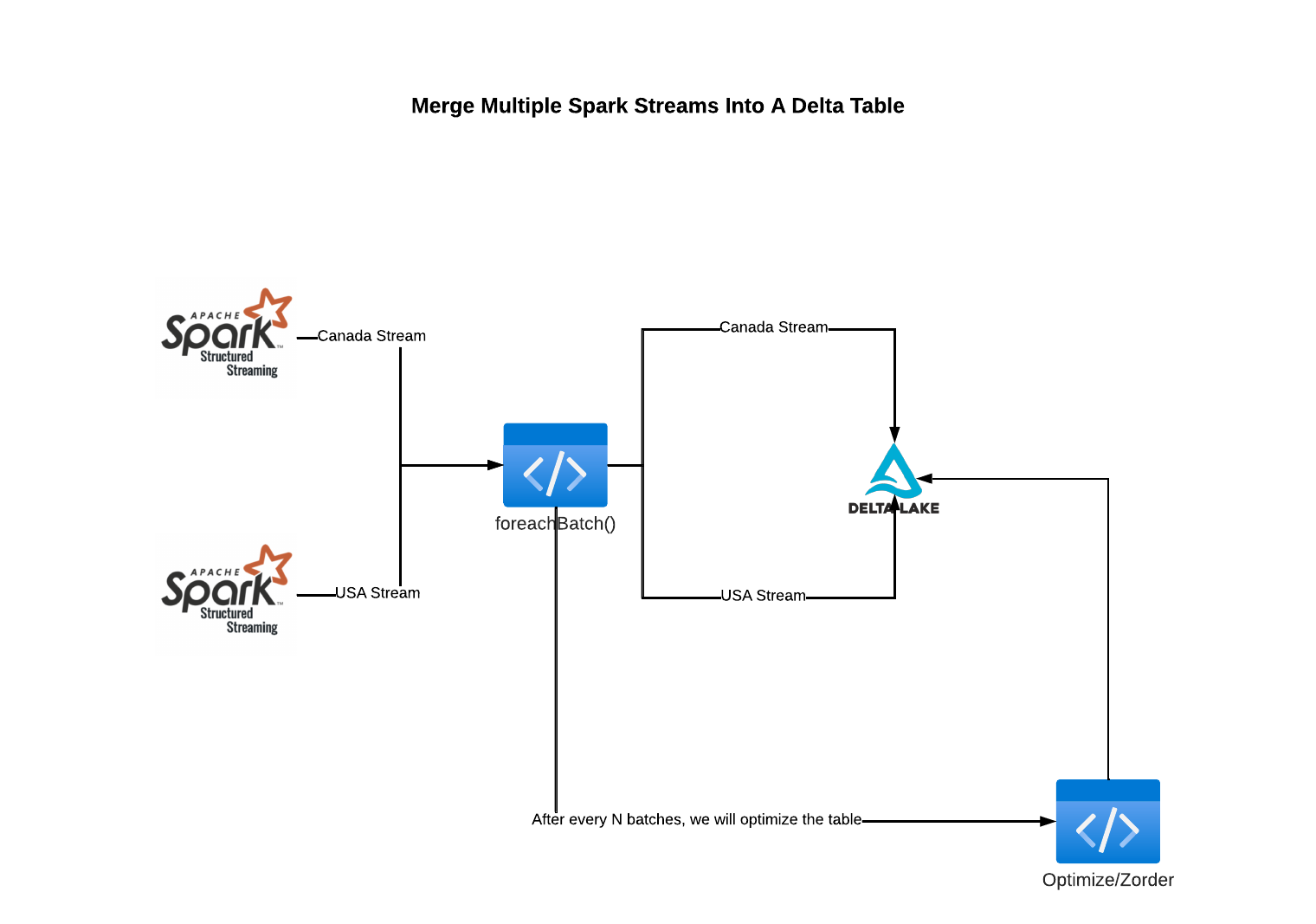# Merge Multiple Spark Streams Into A Delta Table

October 13, 2022

## Merge Multiple Spark Streams Into A Delta Table with working code

This blog will discuss how to read from multiple Spark Streams and merge/upsert data into a single Delta Table. We will also optimize/cluster data of the delta table.

Overall, the process works in the following manner:

1. Read data from a streaming source

2. Use this special function ***foreachBatch. ***Using this we will call any user-defined function responsible for all the processing.

3. Our user-defined function runs the Merge and *Optimize over *the target Delta table.

## ArchitectureFirst, we need some input data to merge. You could technically make a stream out of Kafka, Kinesis, s3, etc.

However, for simplicity we will use .format(’rate’) to generate a stream. Feel free to alter numPartitions & rowsPerSecond . These parameters help you control how much volume of data you want to generate. In the below code, we generated 1,000 rows per second across 100 partitions.

For the purpose of this blog, we will build 2 Spark streams one for each country Canada & USA.

USA’s stream

``````generated_streaming_usa_df = (
.format("rate")
.option("numPartitions", 100)
.option("rowsPerSecond", 1 * 1000)
.selectExpr(
"md5( CAST (value AS STRING) ) as md5"
,"value"
,"value%1000000 as hash"
,"'USA' AS country"
,"current_timestamp() as ingestion_timestamp"
)
)

#display(generated_streaming_usa_df)
``````

``````generated_streaming_canada_df = (
.format("rate")
.option("numPartitions", 100)
.option("rowsPerSecond", 1 * 1000)
.selectExpr(
"md5( CAST (value AS STRING) ) as md5"
,"value"
,"value%1000000 as hash"
,"current_timestamp() as ingestion_timestamp"
)
)

``````

## Parameters / Variables (Feel free to change as per your needs)

``````target_table_name = "to_be_merged_into_table_partitioned_by_country"
check_point_location_for_usa_stream = f"/tmp/delta/{target_table_name}/_checkpoints/_usa/"
join_column_name ="hash"
partition_column = "country"
``````

## Create an Empty Delta table so data could be merged into it

``````#spark.sql(f"""  DROP TABLE IF EXISTS {target_table_name};""")
(
generated_steaming_usa_df.writeStream
.partitionBy(partition_column)
.format("delta")
.outputMode("append").trigger(once=True)
.option("checkpointLocation", check_point_location_for_usa_stream)
.toTable(target_table_name)
)
``````

Check if data is populated. If you do not see any data, just run the above code snippet once more. Sometimes it takes time for the data to show up.

``````display(spark.read.table(target_table_name))
``````

## Now we will build the code for the user-defined function which does all the data processing, merge & Optimize

``````def make_changes_using_the_micro_batch(microBatchOutputDF, batchId: int):
print(f"Processing batchId: {batchId}")
spark_session_for_this_micro_batch = microBatchOutputDF._jdf.sparkSession()
spark_session_for_this_micro_batch.sql(f"""
SELECT *
FROM (
select *
,rank() over(partition by {join_column_name} order by value desc) as dedupe
)
WHERE
dedupe =1
spark_session_for_this_micro_batch.sql(f"""
MERGE INTO {target_table_name} target
on u.{partition_column} = target.{partition_column}
AND u.{join_column_name} = target.{join_column_name}
WHEN MATCHED THEN UPDATE SET *
WHEN NOT MATCHED THEN INSERT *
""")
optimize_every_n_batches = 20
#Define how often should optimize run? for example: at 50, it means that we will run the optimize command every 50 batches of stream data
if batchId % optimize_every_n_batches == 0:
optimize_and_zorder_table(table_name = target_table_name,  zorder_by_col_name = join_column_name)
``````

## Optimize/ Z-order a Delta table

Why do we need to optimize a table? If we keep adding files to our Delta table and never optimize/sort them then over time we need to read a lot of files during merge time. Thus, optimizing the Delta table after every N merges is better. N needs to be decided on your latency requirements. You could start with N as 10 and change it as per your needs.

The below code will run an optimize and zorder command on a given table that is being fed by a stream. Optimize commands can’t run in a silo because it will require us to pause and then resume the stream. Therefore, we need to call this function a part of the upsert function. This enables us to optimize before the next batch of streaming data comes through.

``````from timeit import default_timer as timer

def optimize_and_zorder_table(table_name: str, zorder_by_col_name: str) -> None:
"""
Parameters:
table_name: str
name of the table to be optimized
zorder_by_col_name: str
comma separated list of columns to zorder by. example "col_a, col_b, col_c"
"""
start = timer()
print(f"Met condition to optimize table {table_name}")
sql_query_optimize = f"OPTIMIZE  {table_name} ZORDER BY ({zorder_by_col_name})"
spark.sql(sql_query_optimize)
end = timer()
time_elapsed_seconds = end - start
print(
f"Successfully optimized table {table_name} . Total time elapsed: {time_elapsed_seconds} seconds"
)
``````

## Orchestrate the streaming pipeline end to end

``````(
.writeStream.format('delta')
#.trigger(availableNow=True)
.trigger(processingTime='10 seconds')
.foreachBatch(make_changes_using_the_micro_batch)
.start()
)
``````

Read the USA stream and merge into Delta table

``````(
generated_steaming_usa_df
.writeStream.format('delta')
.trigger(processingTime='10 seconds')
.option("checkpointLocation", check_point_location_for_usa_stream)
.foreachBatch(make_changes_using_the_micro_batch)
.start()
)
``````

Now, let’s validate that data is being populated

``````display(
spark.sql(f"""
SELECT
{partition_column} as partition_column
,count(1) as row_count
FROM
{target_table_name}
GROUP BY
{partition_column}
""")
)
``````

If you have reached so far, you should have an end-to-end pipeline working with streaming data and merging data into a Delta table.